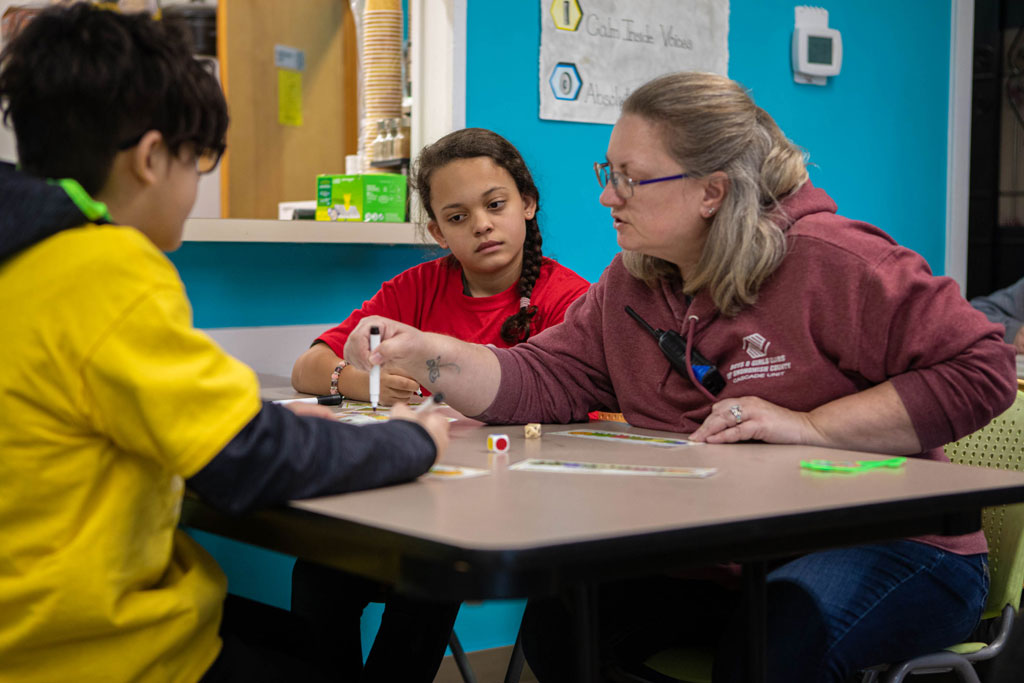# How to Keep Math Stress Free

March 26, 2020

Teaching math can be stressful on the best of days, add uncertainty, multiply in personal attitudes, subtract understanding, and divide by misconceptions and we have a problem.

MANGO Math wants to help!

We are posting across our social media platforms fun, hands-on activities students can do in math. This isn't worksheets or online programs, this is fun exploration of math.

MANGO is actually an acronym of the different math strands:

M - measurement
A - algebraic thinking
N - number sense
G - geometry
O - odds & order (probability & data)

Each week day we will post games, activities and fun problems students can do to explore math and build a strong math understanding.

Feel free to contact me at Mary@mangomath.com if you have questions or suggestions.

If you scroll down you will see our acedemic schedule for families.  Let me know if you would like a PDF copy of that schedule.

## Sample Lessons

Here are a few samples of lessons we will be posting: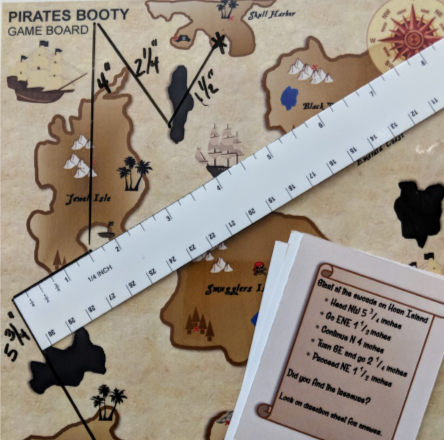Pirates Booty is from our 3rd Grade Kit, students work on linear measurements and fractions.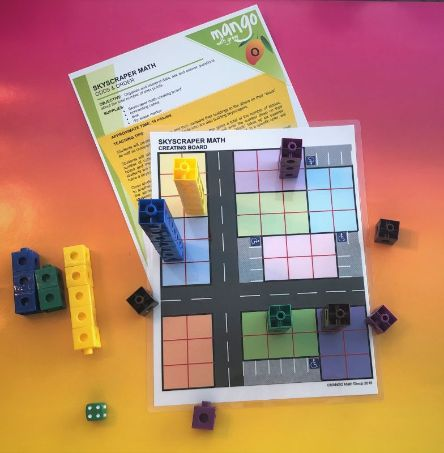Skyscraper Math is from our 1st Grade Kit, students work on the idea of bar graphs.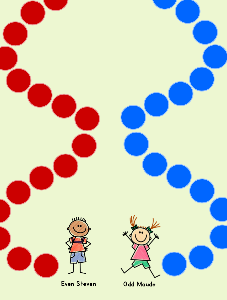Even Steven is from our 2nd Grade Kit, students determine if a sum will be even or odd.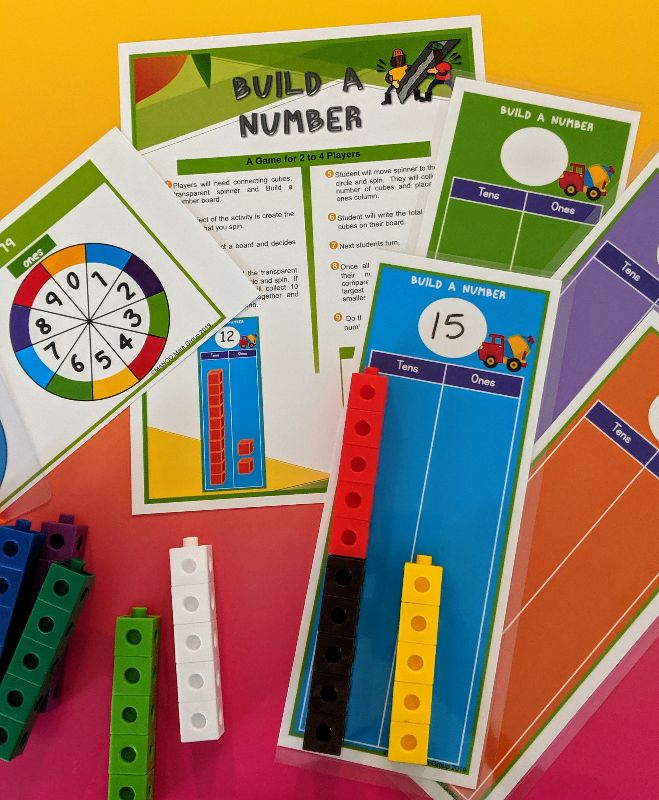Build a Number is from our Kindergarten Kit, students develop an understanding of tens place